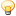A Flash Developer Resource Site

# Thread: Physicsy question: Rebound angle on slope

1. ##Physicsy question: Rebound angle on slope

Hi, I'm new to the forumSo, I've had a tricky question I've had for a while. I've tried everything to solve it but can't quite get it.

I have the xspeed and yspeed of a round ball, xspeed is distance ball moves to the right each frame and yspeed distance ball moves down each frame.

I have 3 different gradients of slopes, one is at 15 degrees off the horizontal.

How can I calculate the xspeed and yspeed of the ball after a collision with, for instance, the 15 degree slope.

Thank you so much for any help, I have been stuck on this for such a long time now.Reply With Quote

2. Wow I need to sleep its way to early, urm I think I know your need, try an inelastic collision in 2 dimensions.
If you get stuck gimme a message and I will give you a hand, anyway 6am = bed.Reply With Quote

3. What you need to do is calculate the angle of incidence. To do this you need to know the normal of the slope and the angle the ball is moving at.

The normal of the slope is the angle of a line pointing perpendicular to the slope. You can get this by adding or subtracting 90 degrees to the angle of the slope. Weather you need to add or subtract depends on the coordinate system you're using, specifically where 0 degrees is.

For example, if 0 degrees indicates a line pointing to the right, positive angles are clockwise, and the ball is approaching the slope from the top, you would need to subtract 90 degrees from the slope's angle.

To find the angle that the ball is traveling at, you need to use some simple trigonometry. Imagine a right triangle defined as follows: Point Z is at the origin (0, 0), point X is at (xspeed, 0) and point Y is at (xspeed, yspeed). Here's a diagram:Now, with 0 degrees defined as pointing to the right (positive x direction) and clockwise rotation, ∠XZY is the angle that the ball is moving at.

To get this angle, use 'Math.atan2(yspeed, xspeed)'. To get the velocity, use the Pythagorean theorem, 'Math.sqrt(yspeed*yspeed+xspeed*xspeed)'

Once you've calculated the angle the ball is moving at, you can add to the slope's normal to get the angle of incidence. According to the law of reflection, the angle of reflection is equal to the angle of incidence. Here's another diagram:For example, if the ball is moving at a 45 degree angle and hits a 15 degree slope, you can calculate the final angle as follows:

final = slope + slope - angle

For more details as to how this formula is derived, consult a physics text. I'd forgotten it and had to muddle through a bunch of not very useful web pages (including some other threads on this forum) to figure it out.

To get the x and y components of the resulting vector, use more trig:

xspeed = velocity * cos(final)
yspeed = velocity * sin(final)

Hope this helps.Reply With Quote

####Posting Permissions

• You may not post new threads
• You may not post replies
• You may not post attachments
• You may not edit your posts
•

 » Home » Movies » Tutorials » Submissions » Board » Links » Reviews » Feedback » Gallery » Fonts » The Lounge » Sound Loops » Sound FX » About FK » Sitemap